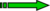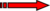# Difference between revisions of "Archer"

## OBJECTIVE: Liquid/Gaz Interface Simulations

Describe the interface motion preciselyLevel Set Method

Handle jump conditions at the interface without artificial smoothingGhost Fluid Method

Respect mass conservationVOF Method

Solve incompressible Navier Stokes equationsProjection MethodArcher is a 3D incompressible Navier Stokes solver with Level Set-Ghost Fluid-VOF coupling and MPI parallelization

## NUMERICS

• Cartesian mesh
• Mac grid (velocities on cell boundaries)
• WENO 5 scheme for convective terms, Adams Bashforth or RK3 or split algorithm in time
• Multigrid algorith for preconditionning Conjugate Gradient Method in Poisson equation solver
• Ghost Fluid method for variable discontinuities at the interface
• CLSVOF for mass conservation
• MPI parallelization

## UNDER DEVELOPMENT

• Immersed boundaries
• Coupling with Lagrangian solver
• New convection scheme (modified Rudman's method)

## GALLERY

### Turbulent jet

Diesel type Jet Atomization : Diameter: 100µm, Liquid velocity 100 ms-1, Turbulence 5%, gas velocity: 0 ms-1, $\rho _{liq}$=696 kgm-3, $\rho _{gas}$=25 kgm-3

$\mu _{liq}$ =1.210-3kgm-1s-1, $\mu _{gas}$=10-5kgm-1s-1, $\sigma$=0.06Nm-1 Numerical simulation by coupling Level Set / VOF / Ghost fluid methods

Mesh : 256x256x2048 (130 millions points) MPI parallelization 128 procs.

### Triple disk injector

• Coupling between internal flow simulations and DNS of primary breakup
• Radius of the jet exit: 90 microns
• Mesh 256x1024x1024 (~270 millions) dx~1.44 micron
• ~3000 nodes on the (half) jet exit
• 2048 procs
• Triple.avi

### Liquid/Gas mixing layer

• Air/water mixing layer
• 30m/s in air, 0.3 in water
• Grid is 512x512x1024 nodes
• Mesh size equals 48 µm.
• 2048 procs, 20 hours for 3500 time steps; Simulation on 70000 time steps, dt~=10-6 s

### Air assisted atomization

• Air/water Jet « Marmottant » Modified Rudman's method two grids
• Ugas=35m/s and Uliq=0.2m/s
• $\rho _{gas}$=1,2 kg/m3 $\rho _{liq}$ =1000 kg/m3
• Lx=45.6mm (4mm+1.7mm)x8 that is 4 the « jet +gas » diameter
• Grid: 256x256x256,(512x512x512 for level set and VOF, mesh is 90µm)
• 1024 procs on Curie, 12h wall-clock for 5000 time steps.
• Simulation is 250000 time steps.
• Marmotte.avi

### 2D gas/liquid shear layer

• M=$\rho _{gas}$$U_{gas}$^2 / $\rho _{liq}$$U_{liq}$^2 = 16
• Ugas=30 m/s Uliq=0.26 m/s
• $\rho _{gas}$=1,2 kg/m3 $\rho _{liq}$ =1000 kg/m3
• Lx=80mm, Ly=40 mm 10mm water + 10mm air + 20mm quiet
• Grid: 1024x512(that is 2048x1024 level set and VOF, mesh is 40 µm)
• 512 procs on Curie
• 18h wall clock for 100000 time steps.
• Simulation on 2 000 000 time steps
• 2Dliquid_shear_layer.avi

### Air assisted atomization

• Air/water Jet « Marmottant » RUDMAN-TYPE Technique with one grid
• Ugas=22.6m/s and Uliq=0.27m/s
• $\rho _{gas}$=1,2 kg/m3 $\rho _{liq}$ =1000 kg/m3
• Lx=34.2mm (4mm+1.7mm)x6 that is 3 x the « jet +gas » diameter
• Grid: 256x256x256,(mesh is 134µm)
• 512 procs on Curie, 12h wall-clock for 30000 time steps.
• Simulation is 800000 time steps.
• Marmotte.avi